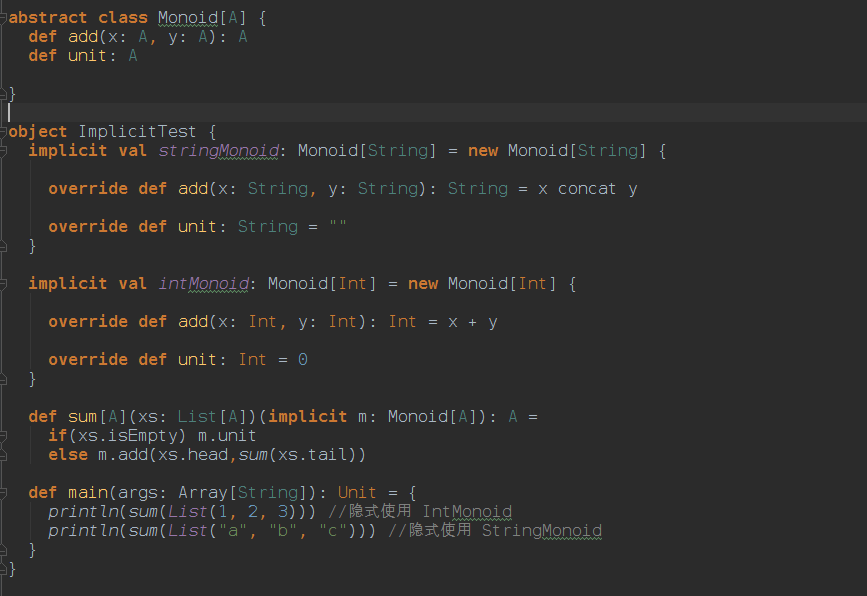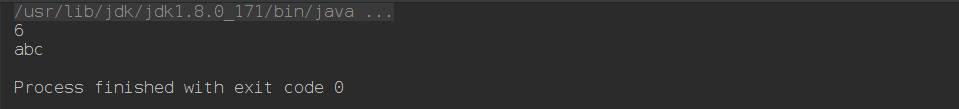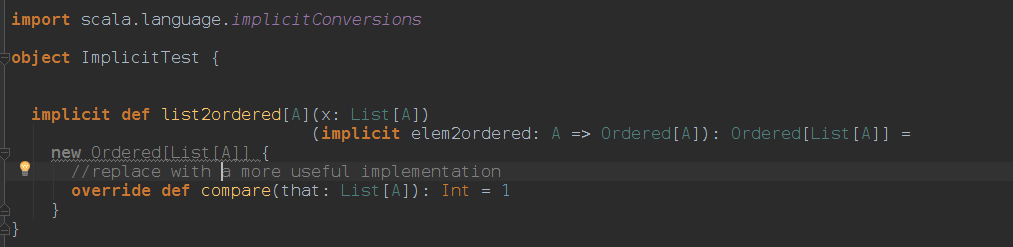# 隐式参数（IMPLICIT PARAMETERS）

Scala 将寻找参数的位置分为两类：

• 在带有隐式参数块的方法被调用时，Scala 首先会查找可以被直接获取（没有前缀）的隐式定义和隐式参数。
• 然后它会查看与隐式候选类型相关联的全部伴生对象（companion objects）中的隐式标记成员。

abstract class Monoid[A] {
def add(x: A, y: A): A
def unit: A
}

object ImplicitTest {
implicit val stringMonoid: Monoid[String] = new Monoid[String] {
def add(x: String, y: String): String = x concat y
def unit: String = ""
}

implicit val intMonoid: Monoid[Int] = new Monoid[Int] {
def add(x: Int, y: Int): Int = x + y
def unit: Int = 0
}

def sum[A](xs: List[A])(implicit m: Monoid[A]): A =
if (xs.isEmpty) m.unit

def main(args: Array[String]): Unit = {
println(sum(List(1, 2, 3)))       // uses IntMonoid implicitly
println(sum(List("a", "b", "c"))) // uses StringMonoid implicitly
}
}Nonoid 定义了一个 add 操作，它组合了一对 A 并返回另一个 A，以及一个称为 unit 的操作能够创建一些特定的 A

intMonoid 是一个可以在 main 函数中被直接获取的隐式定义。它也是正确的类型，所以它会自动地传递给 sum 方法。

6
abc# 隐式转换（IMPLICIT CONVERSIONS）

• 如果表达式 e 是类型 S，并且 S 不符合表达式的预期类型 T
• 在选择带有 S 类型 ee.m 中，如果选择器 m 不表示成员 S

List(1,2,3)  <= List(4,5)

import scala.language.implicitConversions

implicit def list2ordered[A](x: List[A])
(implicit elem2ordered: A => Ordered[A]): Ordered[List[A]] =
new Ordered[List[A]] {
//replace with a more useful implementation
def compare(that: List[A]): Int = 1
}import scala.language.implicitConversions

implicit def int2Integer(x: Int) =
java.lang.Integer.valueOf(x)

• 导入 scala.language.implicitConversions 到隐式转换定义的范围
• 调用编译器时带参数 -language:implicitConversions